### 3.1. Single photon-electron scattering

When a photon is scattered by an electron, the energy and direction of motion of both the photon and the electron are usually altered. The change in properties of the photon is described by the usual Compton scattering formula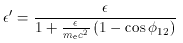(21)

where the electron is assumed to be at rest before the interaction,and' are the photon energies before and after the interaction, and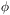12 is the angle by which the photon in deflected in the encounter (see Fig. 3).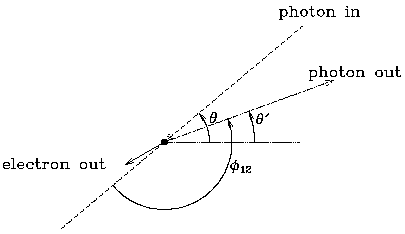Figure 3. The scattering geometry, in the frame of rest of the electron before the interaction. An incoming photon, at angle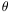relative to the xe axis, is deflected by angle12, and emerges after the scattering at angle' with almost unchanged energy (equation 21). In the observer's frame, where the electron is moving with velocityc along the xe axis, the photon changes energy by an amount depending onand the anglesand' (equation 25).

For low-energy photons and mildly relativistic or non-relativistic electrons,<< me c2 and the scattering is almost elastic (' =). This limit is appropriate for the scatterings in clusters of galaxies that cause the Sunyaev-Zel'dovich effect, and causes a considerable simplification in the physics. Although the scatterings are usually still referred to as inverse-Compton processes, they might better be described as Thomson scatterings in this limit.

In this thermal scattering limit, the interaction cross-section for a microwave background photon with an electron need not be described using the Klein-Nishina formula,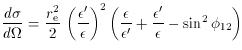(22)

but rather the classical Thomson cross-section formula which results in the limit' ->. Then if the geometry of the collision process in the electron rest frame is as shown in Fig. 3, the probability of a scattering with angleis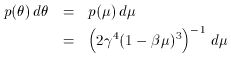(23)

where the electron velocity ve =c, and µ = cos. The probability of a scattering to angle' is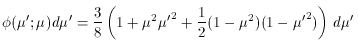(24)

(Chandrasekhar 1950; Wright 1979), and the change of photon direction causes the scattered photon to appear at frequency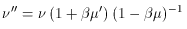(25)

with µ' = cos'.

It is conventional (Wright 1979; Sunyaev 1980; Rephaeli 1995b) to express the resulting scattering in terms of the logarithmic frequency shift caused by a scattering, s (Sunyaev uses u for a related quantity),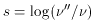(26)

when the probability that a single scattering of the photon causes a frequency shift s from an electron with speedc is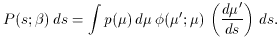(27)

Using (23-25), this becomes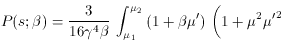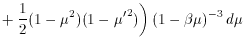(28)

where µ' can be expressed in terms of µ and s as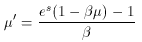(29)

(from equations 25 and 26), and the integral is performed only over real angles, so that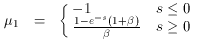(30)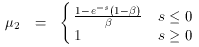(31)

in (28). The integration can be done easily, and Fig. 4 shows the resulting function for several values of. The increasing asymmetry of P (s;) asincreases is caused by relativistic beaming, and the width of the function to zero intensity in s,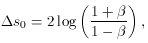(32)

increases because increasingcauses the frequency shift related to a given photon angular deflection to increase.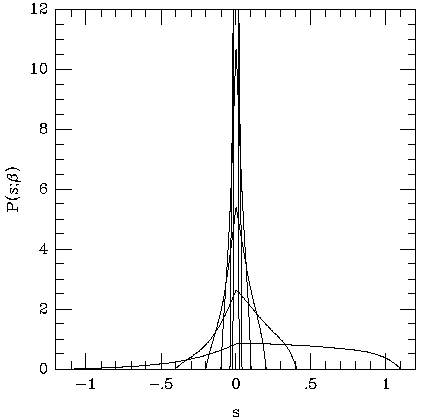Figure 4. The scattering probability function P (s;), for= 0.01, 0.02, 0.05, 0.10, 0.20, and 0.50. The function becomes increasingly asymmetric and broader asincreases.